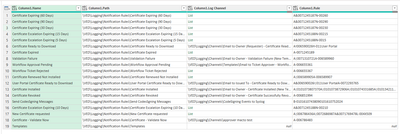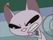cancel
Showing results for
Did you mean:New Member

## Transform list into comma-separated text.

Hi,

My json file allows multivalue in the field, that result in different results in the table.after the import, I can see 'lists' and  the values (for a single value).I struggle to expand all lists, and show all channels in the same cellis it possible to achieve ?

1 ACCEPTED SOLUTIONSolution Sage

Hi, @tall27

``Table.TransformColumns(source_table, {"column_name", (x) => if Value.Type(x) = type list then Text.Combine(List.Transform(x, Text.From), ", ") else x})``Solution Sage

Hi, @tall27

``Table.TransformColumns(source_table, {"column_name", (x) => if Value.Type(x) = type list then Text.Combine(List.Transform(x, Text.From), ", ") else x})``